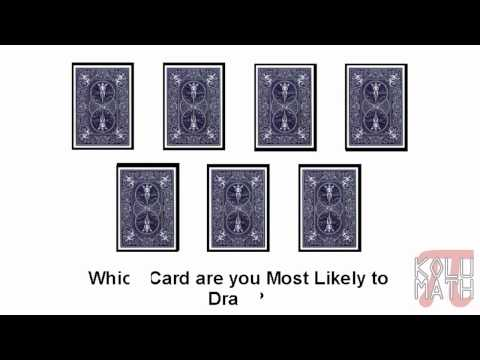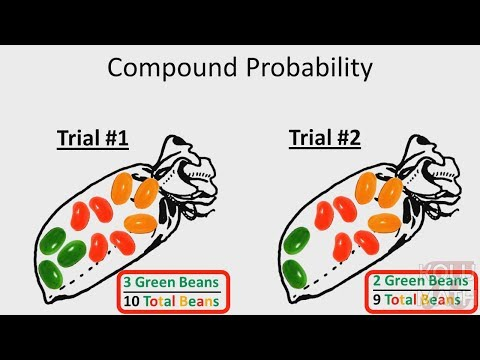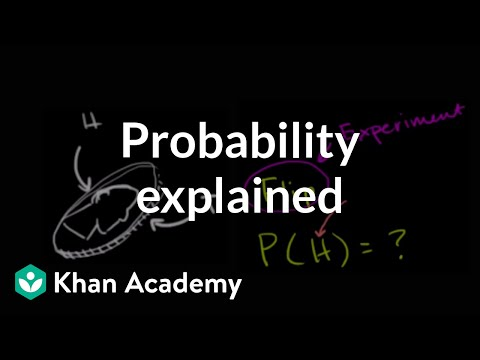## Probability

Subject: Compulsory Maths

Find Your Query

#### Overview

Analysis of certainty or uncertainty of some event is called probability. Experiment, Random experiment, Output, Sample space, Event, Sample point, Elementary point, Equally likely outcomes and Favourable outcomes are defined terms used in probability.

##### ProbabilityProbability is the measure of the likelihood that an event will occur. Probability is quantified as a number between 0 and 1 (where 0 indicates impossibility and 1 indicate certainty). For example,
1. Probability of raining or not raining on a certain day
2. Probability of a student's passing or not an examination
3. The probability of winning or losing a game etc.

Some of the defined terms used in probability are:

Experiment
Observing the outcomes in probability are experiments. For example, tossing a coin to observe whether head or tail turns up is an experiment.

Random Experiment
If the result of an experiment is not certain, then it is called the random experiment.

Outcome
The result obtained in an experiment is called an outcome.

Sample Space
The set of all possible outcomes of an experiment is called sample space.

Event
Any subset of sample space is called an event.

Sample point
Each result or event of a sample space is called sample point. In tossing a coin, H and T are sample points.

Elementary Point
If the numbers of an event are only one then the event is called elementary point

Equally likely outcomes
If there is an equal chance of getting any outcome of an experiment they are equally likely outcomes.

Favourable outcomes
The outcomes of an experiment whose occurrence show the happening of an event is known as favourable events.

For example in tossing a coin ,
P(H) = $\frac{n(H)}{n(S)}$ = $\frac{1}{2}$

P ({H,T}) = $\frac{n (HT) }{n(S)}$ = $\frac{2}{2}$ = 1

General Information of cards, coins and dice on probability:

#### 1. Card

In one packet of the card, there are two colors red and black. Every color has 26 numbers of cards. Red cards are heart and diamond and black cards are spade and club.

In each type of card, there are 1, 2, .......10, J, Q, K which are altogether 13 in number.

Face card: jack (J), queen (Q), and king (K).

In some packets of cards, we find more than 52 cards, which are not necessary for the study of probability.

Drawing a card from the well-shuffled pack of 52 cards, the probability of getting every number is $\frac{1}{52}$.

#### 2. Coins

There are two possible outcomes while tossing a coin. They are head and tail. When the coin is tossed just once, the probability of getting head or tail is equal.

#### 3. Dice

In a dice, there are altogether 6 faces and when the dice is thrown only once, the probability of occurring a number is 1 to 6 equal.

##### Things to remember
• Probability of  event, P(E) =$\frac{the\; number \;of\; favourable\; outcomes}{the\; number\; of\; possible \;outcomes}$ or, P(E) =$\frac{ n(E)}{n(S)}$
• It includes every relationship which established among the people.
• There can be more than one community in a society. Community smaller than society.
• It is a network of social relationships which cannot see or touched.
• common interests and common objectives are not necessary for society.
##### Videos for Probability##### Probability - Part One : Simple Probability##### Probability - Part Two : Compound ProbabilityProbability - Part Two : Compound Probability##### Questions and Answers

Total no. of cards= 52
No. of possible outcomes = 52
No. of ace in a pack of card is 4
No. of favourable outcomes of ace = 4
No. of clubs in a pack of card is 1
No. of favourable outcomes of an ace of clubs = 1
P (ace) = $\frac{No. of favourable outcomes}{No. of possible outcomes}$ = $\frac{4}{52}$ = $\frac{1}{13}$
P (ace of clubs) = $\frac{No. of favourbale outcomes}{No. of possible outcomes}$ = $\frac{1}{52}$

Total no. of marbles = 3 blue + 5 black = 8
No. of possible outcomes = 8
NO, of blue marbles is 3
No. of favourable outcomes of blue marbles= 3
Hence , the probability of getting blue marble ,
P (blue) = $\frac{No. of favourable outcomes}{No. of possible outcomes}$ = $\frac{3}{8}$

Total no. of pages = 260
No. of possible outcomes= m = 260
No. of pages having picture = 13
No. of favourable outcomes = n = 13
No. of pages having no picture = 260 - 13 = 247
(i). Probability of getting page having picture
P (having picture) = $\frac{m}{n}$ = $\frac{13}{260}$ = $\frac{1}{20}$

(ii) P (having no picture) = $\frac{m}{n}$ = $\frac{247}{260}$ = $\frac{19}{20}$Ans.

Total no. of students = 900
No. of possible outcomes = n = 900
No. of girls = 350
No. of boys = (900 - 350) = 550

(i). No. of favourable outcomes = m = 350
Probability that the selected student is a girl ,
P (a girl) = $\frac{m}{n}$ = $\frac{350}{900}$ = $\frac{7}{18}$

(ii). Here , no of favourable outcomes of boys = n = 550
Probability that the selected student is not a girl
P (not girl) = $\frac{m}{n}$ =$\frac{550}{900}$ = $\frac{11}{18}$

Probability of turning up any face = 1
No. of favourable outcomes = 1
Total numbers of faces = 6
No. of possible outcomes = 6
P(turn up any face) = $\frac{no. of favourable outcomes}{no. of favourable outcomes}$ = $\frac{1}{6}$

The faces having even numbers = 2 , 4 , 6
No. of favourable outcomes = 3
Total no. of faces = 6
No. of possible outcomes = 6

P (face having on even no.) = $\frac{no of favourable outcomes}{no of favourable outcomes}$ = $\frac{3}{6}$ = $\frac{1}{2}$

Total no. of faces = 6
No. of possible outcomes = 6
No. of faces that will turn up till = 4
No, of favourable outcomes =4
No. of face that will turn up more 4 = 2
NO. Of favourable outcomes= 2
Now , probability of the faces that will turn up to 4
P (a face that will turn upto4) = $\frac{No of favourable outcomes}{no. of possible outcomes}$
= $\frac{4}{6}$ = $\frac{2}{3}$

Again , probabilty of the faces that will turnup (>4)
P(a face that will turn up (<4) = $\frac{no of favourable outcomes}{no of favourable outcomes}$ = $\frac{2}{6}$ = $\frac{1}{3}$

Here , sample space of all outcomes, H)
S = { ( H , T} , (H , T} {T , H} , {T , T})

(ii). Probability of getting both heads i.e. (H , H) is 1
no, of favourable outcomes = 1
No. of favourable outcomes (i,e members of S) = n(S) = 4
P(H , H) = $\frac{no. of favourable outcomes}{no. of favourable outcomes}$
= $\frac{1}{4}$

(iii). Probability of getting (T , T) = 1
no. of favourbaleoutcomes = 1
no. of possible outcomes = n(S) = 4
probability of getting T in both coins
P (T , T) = $\frac{no of favourbale outcomes }{no of possible outcomes}$ = $\frac{1}{4}$

(iv) . No. of favourable outcomes of getting (H , T) and (T , H) = 2
No. of possible outcomes = n(S) = 4

$\therefore$ P [ (H , T) or (T , H)]b = $\frac{no of favourable outcomes}{no of possible outcomes }$ = $\frac{2}{4}$ = $\frac{1}{2}$

Here , No. of possible outcomes that the number of exactly divisible by 5 - n(S) = 6
Numbers from 1 to 30 that are exactly divisible by 5 are 5 , 10 , , 25 , 30.
No. of 15 , 20 favourable outcomes that rahul will be selected = n(R) = 1

Hence ,
P(R) = $\frac{n(R)}{n(S)}$ = $\frac{1}{6}$ Ans.

Probability that rahulwill not be selected P $\overline {R}$ = 1 - P(R)
= 1 - $\frac{1}{6}$
= $\frac{6 - 1}{6}$
= $\frac{5}{6}$ Ans.

Number of letters in the word is probability = 11
No. of possible outcomes = n(S) = 11
(i). Probability that the card drawn is occuringP =?
No. of favourable outcomes = n(P) = 1
Required probability , P(p) = $\frac{n(P)}{n(S)}$ = $\frac{1}{11}$

(ii) . Probability that the card drawn is occuringb = ?
No. of favourable outcomes n(b) = 2
Required probability P*(b) = $\frac{n(b)}{n(S)}$ = $\frac{2}{11}$

(iii). probability that the card drawn is not occuringb = ?
No. of favourable outcomes n $\overline {b}$ =11 - 2 = 9
Required probability P$\overline {b}$ = $\frac{n (\overline{b})}{n(S)}$ = $\frac{9}{11}$

(iv) probability that the card is not occuring r ,
No. of favourable outcomes n$\overline{r}$ = 11 - 1 = 10
Required probability P($\overline{r}$) = $\frac{\overline{n(r)}}{\overline{n(S)}}$ = $\frac{10}{11}$

(v). Probability that the card drawn is occuringi ,
No. of favourable outcomes n(i) = 2
Required probability P(i) = $\frac{n(i)}{n(S)}$ = $\frac{2}{11}$

Here, total number of experiments = 1200
(i). No. of outcomes occuring 4 =m 193
P(4) = $\frac{total number\;of\;outcomes}{total number\;of\;experiments}$ = $\frac{193}{1200}$

(ii). Number of outcomes which are less than 4 = 186 + 203 + 207 = 596
Total no. of outcomes = 596
P(number less than 4) = $\frac{totalno.\;of\;outcomes}{Total\;no\;of\;experiments}$
= $\frac{596}{1200}$
= $\frac{149}{300}$

(iii). In the above table , sum of frequencies of numbers 5 and 6 which are greater than the no. 4 is 205 + 206 = 411
Total no. of outcomes = 411
P(number greater than 4) = $\frac{total number of outcomes}{total no of experiments}$
= $\frac{411}{1200}$

Here , total no. of born babies = 1000
Total no. of experiments =1000
Number of boys = 514
Total no. of outcomes = 514
Here , probability of newly born baby is a boy ,
P(a boy) = $\frac{total no of outcomes}{total no. of experiments}$ = $\frac{514}{500}$ = $\frac{257}{500}$

Total no. of students = 1000
No. of possible outcomes = 1000
Probability of students whose ages are baove 20 years ,
i.e. P(a student of above 20yrs) = 0.25
No. of favaouable outcomes of student ages are above 20 years.
We know that ,
P ( a student who is above 20yrs) = $\frac{no. of favourbale outcomes}{no of favourbale outcomes}$
or , 0.25 = $\frac{no. of favourable outcomes}{1000}$
or , no. of favourable outcomes = 0.25 $\times$ 1000 = 250

Here , the number of students apperared in SLC exam = 100000
Total no. of experiments = 100000
No. of students who passed in 1stand 2nddivision = 24000
Here , P(passed in 1st and 2nd division) = $\frac{number of favourable outcomes}{total no. of experiments}$
= $\frac{24000}{100000}$
= 0.24

##### Quiz

© 2019-20 Kullabs. All Rights Reserved.